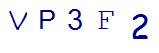# 2nd Grade Common Core Math: Get Comfortable with Math VocabularyLast Updated on August 31, 2021 by Thinkster

Are you ready for 2nd grade common core math? If your child starts bringing home math homework, and you are tasked with the job of helping them, then it’s time for a little refresher course on second grade math vocabulary. Here are some common terms and where they fall in the math spectrum.

### Geometry Terms

In 2nd grade common core math, students begin exploring geometry beyond simple shape recognition, although shapes are still a major part of the curriculum. Here are some terms your child will need to know:

• New shapes: In addition to the triangle, square, circle and rectangle, second-graders need to learn about quadrilaterals, polygons, pentagons, trapezoids, hexagons and octagons. They will also explore the parts of shapes, such as angles, lines, sides, intersections. The introduction of prisms, including spheres, cubes and cones, is also part of this year.
• New terms: This is the year when your child will need to learn the terms parallel, line of symmetry, plane, two-dimensional and three-dimensional
• New descriptions: Your child will need to learn terms like right, left, rectangular, distance, circular, below, beside, above and perimeter to be used to describe the location and shape of different items.

### Measurement and Data

Measuring is a major foundation to 2nd grade common core math. They will be measuring time, money, length, weight, volume, statistics and calendar. Here are some math vocabulary terms to watch for:

• Fractions: Students will need to know what equivalent, equal parts, integer, extend and whole mean in relation to fractions.
• Calendar: Calendar terms will include day, date, week, month, year, event and season.
• Money: Kids will need to know the definitions of the American coins as well as the terms cent and currency.
• Data: Tables, bar graphs, pie charts, tallies, picture graphs, estimates, surveys, predictions, outcomes, symbols and tables all come into play in 2nd grade math.
• Measuring items: Students will need to know metric and American measuring units and the terms weight, volume and length.

### Base Ten Operations

Base ten refers to working with our modern number system, which is based on the value of 10. In 2nd grade math, students will be comparing, counting, adding, subtracting and grouping base ten numbers. This will bring several new terms to add to their math vocabulary, including:

• Number operation terms: sum, addition, subtraction, difference, minus, plus, equals, operation, solve, numeral.
• Place value terms: tens, hundreds, thirds, digit, part, halves and double.
• Grouping terms: estimate, rule pattern, fact families, odds, evens, patterns, classification.
• Comparison terms: small, large, smallest, more than, less than, compare

### Algebraic Terms

No, your second-grader is not going to start algebra, but this is the year that some basic algebra concepts get started. Look for terms like commutative property, expanded form, associative property, unknown, different, alike and sort when it comes to algebra. These are important concepts in Common Core, so you may see them more than you are used to in modern curriculum.

If you find that your child is struggling with any of these basic concepts, help is available. With iPad based tutoring programs, like Thinkster Math, that teach to your child’s level of understanding, you can help your child succeed in these crucial early math concepts, setting the stage for a lifetime of math success.

SummaryArticle Name
2nd Grade Common Core Math: Get Comfortable with Math Vocabulary
Description
If your child is bringing back homework from class, it's probably time for you to brush up too. Here's some vocabulary frequently used in 2nd grade common core math.
Author
Publisher Name
Thinkster Math
Publisher Logo

## Subscribe to Thinkster Blog## Recommended Articles### Unlocking the Code: Teaching Mathematical Symbols and Their Meaning

Knowledge of mathematical symbols is a foundational skill that empowers students to engage with and excel i...